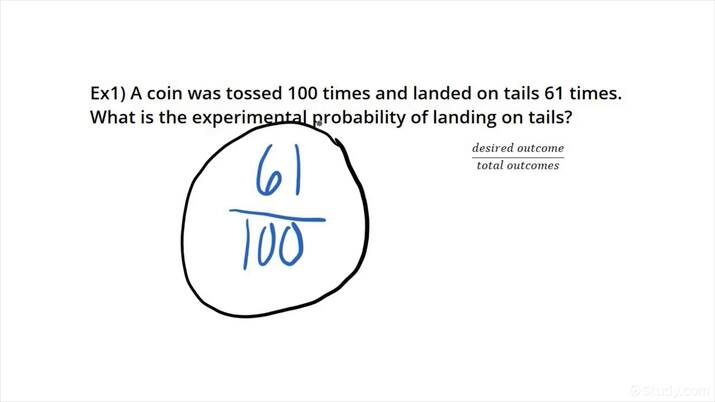# Calculating Experimental ProbabilityAn error occurred trying to load this video.

Try refreshing the page, or contact customer support.

• 00:04 Example 1
Daniel Jibson, AMY MAYERS
• Instructors
Daniel Jibson

Daniel has taught physics and engineering since 2011. He has a BS in physics-astronomy from Brigham Young University and an MA in science education from Boston University. He currently holds a science teaching license for grades 8-12.

•
AMY MAYERS

Amy has taught middle school math and algebra for over seven years. She has a Bachelor's degree in Mathematical Sciences from the University of Houston and a Master's degree in Curriculum and Instruction from The University of St. Thomas. She is a Texas certified teacher for grades 4-12 in Mathematics.

## How to Calculate Experimental Probability

When calculating the probability of an outcome of some event based on experience rather than theoretical methods, follow these steps to correctly compute the probability of an event:

1. Calculate the total number of times the event occurred regardless of outcome.
2. Calculate the total number of times the desired outcome occurred during those events.
3. Divide the total desired outcomes by the total number of event occurrences.
4. Express your probability as a percent.

## Probability Terminology:

Theoretical Probability: The theoretical probability of an outcome is the ideal probability of an event based on mathematical analysis rather than physical analysis. Theoretical probability assumes idealized conditions (that a coin or die is fair, for example, and has an equal chance of landing on any side).

Experimental Probability: The experimental probability of an outcome is the actual probability of the occurrence based on observational data rather than theoretical mathematical analysis. This method determines the probability of the actual event which may not necessarily be perfectly fair. Experimental probability is determined as the ratio of the number of desired outcomes to the number of total occurrences.

The following two problems demonstrate how to determine the experimental probability of an event.

## Calculating Experimental Probability: Example Problem 1

A die is rolled 100 times and the following outcomes are recorded:

 Side Times Rolled 1 27 2 5 3 17 4 22 5 14 6 15

Determine the experimental probability of rolling an odd number with this die.

We know that the event occurred 100 times. To determine the number of desired outcomes, we will add the number of odd-number occurrences: {eq}27 + 17 + 14 = 58 {/eq}

Therefore, the probability of rolling an odd number with this die is experimentally the ratio of these two:

$$P(\rm{odd}) = \dfrac{58}{100} = 0.58$$

We will multiply this number by 100 to express it as a percentage:

$$0.58 \times 100 = 58\%$$

With this (not fair) die, there is a 58% chance of rolling an odd number.

## Calculating Experimental Probability: Example Problem 2

After a night of bowling, the scores of every bowler in the building were tallied up:

 Score Range Number of Games 0-50 6 51-100 22 101-150 30 151-200 15 201-250 5 251-300 2

What is the experimental probability of bowling a game better than 200 on this night?

In this case, there is basically no way to even calculate theoretical probabilities of a night of bowling since it depends on so many different, unpredictable factors. The experimental odds are the only way of determining an approximation of the odds.

The total number of games bowled is {eq}6+22+30+15+5+2 = 80 {/eq}

And the total number of games above 200 is: {eq}5+2 =7 {/eq}

Therefore, the experimental probability of bowling a game above 200 is:

$$P(>200) = \dfrac{7}{80} = 0.0875$$

There was an 8.75% chance of bowling a game above 200 at this bowling alley on that night. Please note that this is not the odds of bowling a 200+ for any other circumstance as the game count that night depended upon who was there, their skill level, and their luck that night. A new group of people might produce entirely different results.

Get access to thousands of practice questions and explanations!

Support